## Monday, August 5, 2013

### Creating vectorial isobands with Python

GDAL has an utility program, gdal_contour, that generates contour lines from a raster file (take a look at his example post). GDAL provides access to this method through its API, so contour lines can be generated inside your GDAL python scripts.

Unfortunately, there is no way to generate isobands, that is, filled contour lines that use polygons instead of polylines. Creating isobands allows the creation of nice contour maps easily, colouring the zones between two values:Using contour lines, the result is this one:

Both visualizations are drawn directly from QGIS.

So I created two scripts to get the result. As usual, you can download them from my GitHub account.
I created two scripts, since the one I like the most uses matplotlib, so sometimes it's not convenient to install. On the other hand, the other has less requirements (GDAL python), but the code is dirtier.

Both have the same usage, which is very similar to the gdal_contour usage:

    Usage: isobands_matplotlib.py [-h] [-b band] [-off offset] [-i interval]
[-nln layer_name] [-a attr_name] [-f formatname]
src_file out_file
Calculates the isobands from a raster into a vector file

positional arguments:
src_file         The raster source file
out_file         The vectorial out file
optional arguments:
-h, --help       show this help message and exit
-b band          The band in the source file to process (default 1)
-off offset      The offset to start the isobands (default 0)
-i interval      The interval (default 0)
-nln layer_name  The out layer name (default bands)
-a attr_name     The out layer attribute name (default h)
-f formatname    The output file format name (default ESRI Shapefile)
Just choose the input and output files, the input file band to process (1 by default), and the interval, and you will get the  vector file in the GIS format of your choice.

Now, the code of both script, step by step.

### isobands_matplotlib.py

As it's name suggests,  this version uses matplotlib, and more specifically the contourf function, to create the isobands.

The script has a function called isobands, that actually generates the data, and a main part that reads the user input values:
if __name__ == "__main__":

PARSER = ArgumentParser(
description="Calculates the isobands from a raster into a vector file")
PARSER.add_argument("src_file", help="The raster source file")
PARSER.add_argument("out_file", help="The vectorial out file")
help="The band in the source file to process (default 1)",
type=int, default = 1, metavar = 'band')
help="The offset to start the isobands (default 0)",
type=float, default = 0.0, metavar = 'offset')
help="The interval  (default 0)",
type=float, default = 0.0, metavar = 'interval')
help="The out layer name  (default bands)",
default = 'bands', metavar = 'layer_name')
help="The out layer attribute name  (default h)",
default = 'h', metavar = 'attr_name')
help="The output file format name  (default ESRI Shapefile)",
default = 'ESRI Shapefile', metavar = 'formatname')
ARGS = PARSER.parse_args()

isobands(ARGS.src_file, ARGS.b, ARGS.out_file, ARGS.f, ARGS.nln, ARGS.a,
ARGS.off, ARGS.i)

I used the argparse.ArgumentParser to read the script arguments, since is easy to use and it should come woth most of the updated python distributions (since python 2.7, I think).
This part of the code is quite easy to understand, it simply sets the arguments with their default value, sets if they are mandatory or not, etc.

The isobands function creates the vector file:
def isobands(in_file, band, out_file, out_format, layer_name, attr_name,
offset, interval, min_level = None):
'''
The method that calculates the isobands
'''
ds_in = gdal.Open(in_file)
band_in = ds_in.GetRasterBand(band)
xsize_in = band_in.XSize
ysize_in = band_in.YSize

geotransform_in = ds_in.GetGeoTransform()

srs = osr.SpatialReference()
srs.ImportFromWkt( ds_in.GetProjectionRef() )

#Creating the output vectorial file
drv = ogr.GetDriverByName(out_format)

if exists(out_file):
remove(out_file)
dst_ds = drv.CreateDataSource( out_file )

dst_layer = dst_ds.CreateLayer(layer_name, geom_type = ogr.wkbPolygon,
srs = srs)

fdef = ogr.FieldDefn( attr_name, ogr.OFTReal )
dst_layer.CreateField( fdef )

x_pos = arange(geotransform_in,
geotransform_in + xsize_in*geotransform_in, geotransform_in)
y_pos = arange(geotransform_in,
geotransform_in + ysize_in*geotransform_in, geotransform_in)
x_grid, y_grid = meshgrid(x_pos, y_pos)

raster_values = band_in.ReadAsArray(0, 0, xsize_in, ysize_in)

stats = band_in.GetStatistics(True, True)
if min_level == None:
min_value = stats
min_level = offset + interval * floor((min_value - offset)/interval)

max_value = stats
#Due to range issues, a level is added
max_level = offset + interval * (1 + ceil((max_value - offset)/interval))

levels = arange(min_level, max_level, interval)

contours = plt.contourf(x_grid, y_grid, raster_values, levels)

for level in range(len(contours.collections)):
paths = contours.collections[level].get_paths()
for path in paths:

feat_out = ogr.Feature( dst_layer.GetLayerDefn())
feat_out.SetField( attr_name, contours.levels[level] )
pol = ogr.Geometry(ogr.wkbPolygon)

ring = None

for i in range(len(path.vertices)):
point = path.vertices[i]
if path.codes[i] == 1:
if ring != None:
ring = ogr.Geometry(ogr.wkbLinearRing)

feat_out.SetGeometry(pol)
if dst_layer.CreateFeature(feat_out) != 0:
print "Failed to create feature in shapefile.\n"
exit( 1 )

feat_out.Destroy()

• Lines 26 to 49 open the input file and create the output vector file.
• Lines 53 to 57 set two matrices, containing the coordinates for each pixel in the input file. To do it, two vectors are created first with the coordinates calculated from the input geotransform, and then the meshgrid is called to generate the 2d matrices, needed by matplotlib to make its calculations.
• Lines 59 to 70 read the input file data and calculates the levels inside it, from the interval and the offset. Since calculating isobands for levels not present in the file isn't efficient, the minimum and maximum values in the input file are calculated before the isobands.
• The line 72 is where the isobands are actually calculated using the contourf function.
•  Lines 76 to 105 create the polygons at the output vector file.
• The generated contour has a property called collections, containing every isoband.
• Each element in the collections has the paths of the isolines.
• The property codes in the path indicates if a new ring has to be generated.
• The other lines are to generate an OGR feature

### isobands_gdal.py

This script is quite dirtier, since I have used a trick to get the isobands from the ContourGenerate function.
Closing the contour lines, which is what has to be done to transform isolines to isobands, is not an easy task.
Looking at some D3js examples I got the idea from Jason Davies conrec.js. I can't find the original example now, but basicaly, the idea is to add some pixels around the image with a value lower than the lowest value, so all the isolines will be closed. The solution gets closer, but some problems must be solved:
• Convert the isolines to polygons
• Clip the vectors to the original extent
• Substract the overlapping polygons, since when the lines are converted to polygons, all the area above the isoline value is contained in the polygon even though other lines with higher values are inside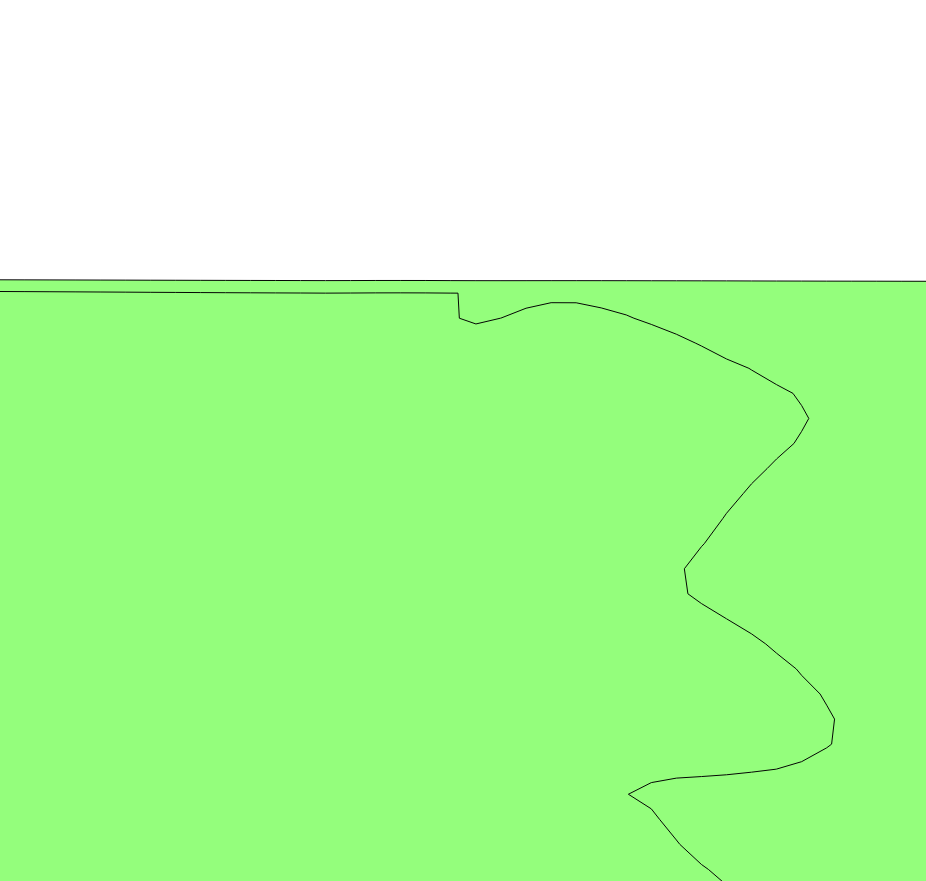When not clipped, the isolines will create strange effects at the borders

The main part code is exacly the same as in the other script, and the isobands function is:

def isobands(in_file, band, out_file, out_format, layer_name, attr_name,
offset, interval, min_level = None):
'''
The method that calculates the isobands
'''
ds_in = gdal.Open(in_file)
band_in = ds_in.GetRasterBand(band)
xsize_in = band_in.XSize
ysize_in = band_in.YSize

stats = band_in.GetStatistics(True, True)

if min_level == None:
min_value = stats
min_level = ( offset + interval *
(floor((min_value - offset)/interval) - 1) )
nodata_value = min_level - interval

geotransform_in = ds_in.GetGeoTransform()

srs = osr.SpatialReference()
srs.ImportFromWkt( ds_in.GetProjectionRef() )

data_in = band_in.ReadAsArray(0, 0, xsize_in, ysize_in)

#The contour memory
contour_ds = ogr.GetDriverByName('Memory').CreateDataSource('')
contour_lyr = contour_ds.CreateLayer('contour',
geom_type = ogr.wkbLineString25D, srs = srs )
field_defn = ogr.FieldDefn('ID', ogr.OFTInteger)
contour_lyr.CreateField(field_defn)
field_defn = ogr.FieldDefn('elev', ogr.OFTReal)
contour_lyr.CreateField(field_defn)

#The in memory raster band, with new borders to close all the polygons
driver = gdal.GetDriverByName( 'MEM' )
xsize_out = xsize_in + 2
ysize_out = ysize_in + 2

column = numpy.ones((ysize_in, 1)) * nodata_value
line = numpy.ones((1, xsize_out)) * nodata_value

data_out = numpy.concatenate((column, data_in, column), axis=1)
data_out = numpy.concatenate((line, data_out, line), axis=0)

ds_mem = driver.Create( '', xsize_out, ysize_out, 1, band_in.DataType)
ds_mem.GetRasterBand(1).WriteArray(data_out, 0, 0)
ds_mem.SetProjection(ds_in.GetProjection())
#We have added the buffer!
ds_mem.SetGeoTransform((geotransform_in-geotransform_in,
geotransform_in, 0, geotransform_in-geotransform_in,
0, geotransform_in))
gdal.ContourGenerate(ds_mem.GetRasterBand(1), interval,
offset, [], 0, 0, contour_lyr, 0, 1)

#Creating the output vectorial file
drv = ogr.GetDriverByName(out_format)
if exists(out_file):
remove(out_file)
dst_ds = drv.CreateDataSource( out_file )

dst_layer = dst_ds.CreateLayer(layer_name,
geom_type = ogr.wkbPolygon, srs = srs)

fdef = ogr.FieldDefn( attr_name, ogr.OFTReal )
dst_layer.CreateField( fdef )

geometry_list = {}
for feat_in in contour_lyr:
value = feat_in.GetFieldAsDouble(1)

geom_in = feat_in.GetGeometryRef()
points = geom_in.GetPoints()

if ( (value >= min_level and points == points[-1]) and
(points == points[-1]) ):
if (value in geometry_list) is False:
geometry_list[value] = []

pol = ogr.Geometry(ogr.wkbPolygon)
ring = ogr.Geometry(ogr.wkbLinearRing)

for point in points:

p_y = point
p_x = point

if p_x < (geotransform_in + 0.5*geotransform_in):
p_x = geotransform_in + 0.5*geotransform_in
elif p_x > ( (geotransform_in +
(xsize_in - 0.5)*geotransform_in) ):
p_x = ( geotransform_in +
(xsize_in - 0.5)*geotransform_in )
if p_y > (geotransform_in + 0.5*geotransform_in):
p_y = geotransform_in + 0.5*geotransform_in
elif p_y < ( (geotransform_in +
(ysize_in - 0.5)*geotransform_in) ):
p_y = ( geotransform_in +
(ysize_in - 0.5)*geotransform_in )

geometry_list[value].append(pol)

values = sorted(geometry_list.keys())

geometry_list2 = {}

for i in range(len(values)):
geometry_list2[values[i]] = []
interior_rings = []
for j in range(len(geometry_list[values[i]])):
if (j in interior_rings) == False:
geom = geometry_list[values[i]][j]

for k in range(len(geometry_list[values[i]])):

if ((k in interior_rings) == False and
(j in interior_rings) == False):
geom2 = geometry_list[values[i]][k]

if j != k and geom2 != None and geom != None:
if geom2.Within(geom) == True:

geom3 = geom.Difference(geom2)
interior_rings.append(k)
geometry_list[values[i]][j] = geom3

elif geom.Within(geom2) == True:

geom3 = geom2.Difference(geom)
interior_rings.append(j)
geometry_list[values[i]][k] = geom3

for j in range(len(geometry_list[values[i]])):
if ( (j in interior_rings) == False and
geometry_list[values[i]][j] != None ):
geometry_list2[values[i]].append(geometry_list[values[i]][j])

for i in range(len(values)):
value = values[i]
if value >= min_level:
for geom in geometry_list2[values[i]]:

if i < len(values)-1:

for geom2 in geometry_list2[values[i+1]]:
if geom.Intersects(geom2) is True:
geom = geom.Difference(geom2)

feat_out = ogr.Feature( dst_layer.GetLayerDefn())
feat_out.SetField( attr_name, value )
feat_out.SetGeometry(geom)
if dst_layer.CreateFeature(feat_out) != 0:
print "Failed to create feature in shapefile.\n"
exit( 1 )
feat_out.Destroy()

• Lines 23 to 44 read the input file, including the data, geotransform, etc.
• Lines 47 to 54 create a vector file where the contour lines will be written. Since the data must be clipped later, the file is a temporary memory file.
• Lines 56 to 71 create a memory raster  file with the input data, plus a buffer around it with a value lower than the lowest in the input data, so the contour lines will get closed.
• Line 74 calls the ContourGenerate function, that will add the contour lines to the vectorial memory file.
• Lines 77 to 91 create the actual output file
• Lines 93 to 130 create a collection of geometries with the polygons.
• The lines are transformed to polygons by creating a ring from the polyline and adding it to a polygon.
• Lines 113 to 123 clip the polygons to the original geotransform, by moving the coordinates of the points outside the geotransform to the border.
• Lines 138 to 167 stores the difference from the polygons that overlap, so the output polygons contain only the zones where the values are in the interval.
• Lines 170 to 187 write the final polygons to the output file, the same way than the other script.

### Notes:

An other option would be, of course, creating the polygons myself. I tried to do it, but as you can see at the marching squares wikipedia page, the algorithm is not easy when using polygons, and especially the matplotlib version of the scripts is quite clean.

The scripts have a problem when there is a nodata zone, since I haven't added the code to handle it.

Finally, as asked in the GDAL-dev mailing list, the best option would be modifying the ContourGenerate function to create isobands, but I think that is out of my scope.
The discussion at the GDAL-dev mailing list where I posted the first version.

The data used for the examples is the same I used at the raster classification post.

Some links I have used:
http://matplotlib.org/api/path_api.html
http://matplotlib.org/api/pyplot_api.html#matplotlib.pyplot.contourf
http://matplotlib.org/basemap/api/basemap_api.html#mpl_toolkits.basemap.Basemap.contourf

1.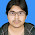Hi, how nice your services. I am very interested that. More services you are provided to us and personal thanks for that. I like your services. I am happy for your clipping path services and we are satisfied of our services. Nice site about clipping path and you are work professionally. For more about clipping path

2.Hi Veciana - just used this script today in some transport analysis work I was doing, and it worked a treat. Nice one!

(Though I found the GDAL version ran considerably slower than the one that uses Matplotlib).

3.I can't even express how amazing your blog is, if it wasn't for your hard work I don't think any novice FOSS geospatial analyst would get anything done.

4.BTW, here's a link to one of the Isoband shapes I created using the script, based on averaging some raster outputs from the OpenTripPlanner tool:
https://www.dropbox.com/s/yyzo7cw00zkk0au/LygonSt-ave-0800.png

5.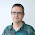Nice example Pat! I'm happy to know that somebody is using it.

6.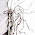Hi, nice idea!! I have been looking for a function to polyonize a raster , in which pixel values represent classes. That is by far the best I have seen.
For my data I can only make the matplotlib option. My testdata is raster with pixel values from 1 to 14, each one means a landcover class.
I set initial an interval of 1 and it worked great, but for adjacent pixels with consecutive values, e.g 1 and 2, the function fails to split them and groups them together, so instead of tow isobands, only one is created.
I have been playing around with your scritpt and it seems that countourf is the reason, because contour (lines) can draw correctly the lines but contourf cannot get the right isobands.
I set an inteval of 0.999 and then works perfectly!
Thanks!!

1.Thank you.
Actually, what would be great is adding the functionality directlyto GDAL so it can be used with all the usual software

7.Images Retouch is a professional Clipping Path Service Company and provides Clipping Path Services, Images Retouching Services and more for additional details http://www.imagesretouch.com

8.Great job. This script really helped me out!
I can recommend to use the matplotlib because it handles the nodata values better and creates single polygons while gdal creates very large multipolygons.
I also recommend to change the band_in.GetStatistics(True, True) to band_in.GetStatistics(False, True) because approximated statistic can cause wrong min/max values when you work with larger raster datasets.
Cheers!

9.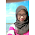hi, i have precipitation data and i plot it using matplotlib, but i want to get shapefile as the output. do you know how to get a shapefile as matplotlib output?